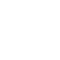# One, Two and Three Dimension Flow

One dimensional flow is the type of flow in which the flow characteristics such as velocity, pressure, density, temperature etc. are the function of time and one space co-ordinate only.

Mathematically,

u=f(x) ; v=0 ; w=0… for steady flow
u=f(x) ; v=0 ; w=0… for unsteady flow

where u, v and w are velocity components in x, y and z directions respectively.

##### Examples:

Flow in a pipe where the average flow parameters are considered for analysis.

Two dimensional flow is the type of flow in which the flow characteristics are the function of time and two rectangular space co-ordinates.

Mathematically,

u=f1(x,y)
v=f2(x,y)
u=f1(x,y,t)
v=f2(x,y,t)

##### Examples:
• Flow between parallel plates of infinite extent.
• Flow in main stream of a wide river.

Three dimensional flow is a type of flow in which the flow characteristics are the function of time and three mutually perpendicular directions.

Mathematically,

u=f1(x,y,z); v=f2(x,y,z); w=f3(x,y,z) for steady flow
u=f1(x,y,z,t); v=f2(x,y,z,t); w=f3(x,y,z,t) for unsteady flow

##### Examples:
• Flow in a converging or diverging pipe or channel.
• Flow in an open channel in which the width and the water depth are of the same order of magnitude.
1. See More : Float technique
2. See More : What is a mobile robots?
3. See More : High energy rate forming### About the author#### Santhakumar Raja

Hi, This blog is dedicated to students to stay update in the education industry. Motivates students to become better readers and writers.

View all posts